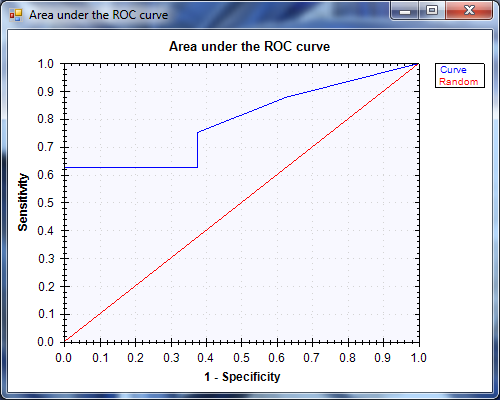Inheritance Hierarchy
SystemObject

Namespace:  Accord.Statistics.Analysis
Assembly:  Accord.Statistics (in Accord.Statistics.dll) Version: 3.8.0Syntax
```[SerializableAttribute]

The ReceiverOperatingCharacteristic type exposes the following members.Constructors
NameDescriptionReceiverOperatingCharacteristic(Boolean, Int32)
Constructs a new Receiver Operating Characteristic modelReceiverOperatingCharacteristic(Double, Double)
Constructs a new Receiver Operating Characteristic modelReceiverOperatingCharacteristic(Int32, Double)
Constructs a new Receiver Operating Characteristic model
TopProperties
NameDescriptionActual
Gets the actual values given by the test.Area
Gets the area under this curve (AUC).Expected
Gets the ground truth values, or the values which should have been given by the test if it was perfect.NegativeAccuracies
Gets DeLong's pseudoaccuracies for the negative subjectsNegativeResults
Gets the actual test results for subjects which should have been labeled as negative.Negatives
Gets the number of actual negative cases.Observations
Gets the number of cases (observations) being analyzed.Points
Gets the points of the curve.PositiveAccuracies
Gets DeLong's pseudoaccuracies for the positive subjects.PositiveResults
Gets the actual test results for subjects which should have been labeled as positive.Positives
Gets the number of actual positive cases.StandardError
Gets the standard error for the Area.Variance
Gets the variance of the curve's Area.
TopMethods
NameDescriptionCompute(Double)
Computes a ROC curve with 1/increment pointsCompute(Double)
Computes a ROC curve with the given increment pointsCompute(Int32)
Computes a n-points ROC curve.Compute(Double, Boolean)
Computes a ROC curve with 1/increment pointsComputePoint
Computes a single point of a ROC curve using the given cutoff value.Equals
Determines whether the specified object is equal to the current object.
(Inherited from Object.)Finalize
Allows an object to try to free resources and perform other cleanup operations before it is reclaimed by garbage collection.
(Inherited from Object.)GetHashCode
Serves as the default hash function.
(Inherited from Object.)GetScatterplot
Generates a Scatterplot representing the ROC curve.GetType
Gets the Type of the current instance.
(Inherited from Object.)Load(Stream)
Loads a curve from a stream.Load(String)
Loads a curve from a file.MemberwiseClone
Creates a shallow copy of the current Object.
(Inherited from Object.)Save(Stream)
Saves the curve to a stream.Save(String)
Saves the curve to a stream.ToString
Returns a String that represents this curve.
(Overrides ObjectToString.)
TopExtension Methods
NameDescriptionHasMethod
Checks whether an object implements a method with the given name.
(Defined by ExtensionMethods.)IsEqual
Compares two objects for equality, performing an elementwise comparison if the elements are vectors or matrices.
(Defined by Matrix.)To(Type)Overloaded.
Converts an object into another type, irrespective of whether the conversion can be done at compile time or not. This can be used to convert generic types to numeric types during runtime.
(Defined by ExtensionMethods.)ToTOverloaded.
Converts an object into another type, irrespective of whether the conversion can be done at compile time or not. This can be used to convert generic types to numeric types during runtime.
(Defined by ExtensionMethods.)
TopRemarks

In signal detection theory, a receiver operating characteristic (ROC), or simply ROC curve, is a graphical plot of the sensitivity vs. (1 − specificity) for a binary classifier system as its discrimination threshold is varied.

This package does not attempt to fit a curve to the obtained points. It just computes the area under the ROC curve directly using the trapezoidal rule.

Also note that the curve construction algorithm uses the convention that a higher test value represents a positive for a condition while computing sensitivity and specificity values.

References:

• Anaesthesist. The magnificent ROC. Available on: http://www.anaesthetist.com/mnm/stats/roc/Findex.htmExamples

The following example shows how to measure the accuracy of a binary classifier using a ROC curve.

```// This example shows how to measure the accuracy of a
// binary classifier using a ROC curve. For this example,
// we will be creating a Support Vector Machine trained
// on the following training instances:

double[][] inputs =
{
// Those are from class -1
new double[] { 2, 4, 0 },
new double[] { 5, 5, 1 },
new double[] { 4, 5, 0 },
new double[] { 2, 5, 5 },
new double[] { 4, 5, 1 },
new double[] { 4, 5, 0 },
new double[] { 6, 2, 0 },
new double[] { 4, 1, 0 },

// Those are from class +1
new double[] { 1, 4, 5 },
new double[] { 7, 5, 1 },
new double[] { 2, 6, 0 },
new double[] { 7, 4, 7 },
new double[] { 4, 5, 0 },
new double[] { 6, 2, 9 },
new double[] { 4, 1, 6 },
new double[] { 7, 2, 9 },
};

int[] outputs =
{
-1, -1, -1, -1, -1, -1, -1, -1, // fist eight from class -1
+1, +1, +1, +1, +1, +1, +1, +1  // last eight from class +1
};

// Next, we create a linear Support Vector Machine with 4 inputs
SupportVectorMachine machine = new SupportVectorMachine(inputs: 3);

// Create the sequential minimal optimization learning algorithm
var smo = new SequentialMinimalOptimization(machine, inputs, outputs);

// We learn the machine
double error = smo.Run();

// And then extract its predicted labels
double[] predicted = new double[inputs.Length];
for (int i = 0; i < predicted.Length; i++)
predicted[i] = machine.Compute(inputs[i]);

// At this point, the output vector contains the labels which
// should have been assigned by the machine, and the predicted
// vector contains the labels which have been actually assigned.

// Create a new ROC curve to assess the performance of the model
var roc = new ReceiverOperatingCharacteristic(outputs, predicted);
roc.Compute(100); // Compute a ROC curve with 100 cut-off points

// Generate a connected scatter plot for the ROC curve and show it on-screen
ScatterplotBox.Show(roc.GetScatterplot(includeRandom: true), nonBlocking: true)

.SetSymbolSize(0)      // do not display data points
.SetLinesVisible(true) // show lines connecting points
.SetScaleTight(true)   // tighten the scale to points
.WaitForClose();```

The resulting graph is shown below.See Also